#### You may also like### Chess

What would be the smallest number of moves needed to move a Knight from a chess set from one corner to the opposite corner of a 99 by 99 square board?### 2001 Spatial Oddity

With one cut a piece of card 16 cm by 9 cm can be made into two pieces which can be rearranged to form a square 12 cm by 12 cm. Explain how this can be done.### Screwed-up

A cylindrical helix is just a spiral on a cylinder, like an ordinary spring or the thread on a bolt. If I turn a left-handed helix over (top to bottom) does it become a right handed helix?

# Making Rectangles, Making Squares

##### Age 11 to 14 Challenge Level:

Lu of St Peter's RC Primary School, sent us the following working:

I use two equilateral triangles and two isosceles triangles to make a basic rectangle.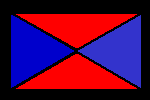I have $20$ equilateral triangles and $20$ isosceles triangles, so I can make $10$ basic rectangles.

I can make:

1 row of $10$ basic rectangles long side down ($1$ row of $9, 8, 7, 6, 5, 4, 3, 2$ basic rectangles as well).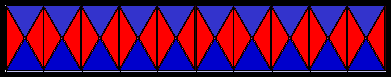$2$ rows of $5$ basic rectangles ($2$ rows of $4, 3, 2, 1$ basic rectangles as well).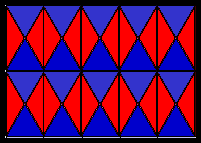$1$ row of $10$ basic rectangles ($1$ row of $9, 8, 7, 6, 5, 4, 3, 2$ basic rectangles as well).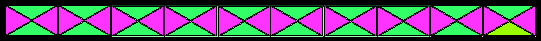$2$ rows of $5$ basic rectangles ($2$ rows of $4, 3, 2, 1$ basic rectangles as well).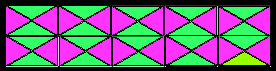$3$ rows of $3$ basic rectangles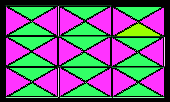Totally I have made $31$ rectangles.